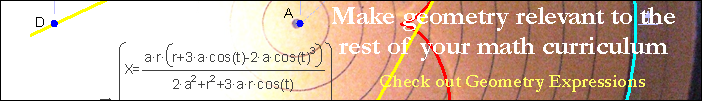Segment Area in Radians

Download Math Illustrations File

The area of a segment of a circle is the sector area decreased by the area of the triangle formed by the chord and two radii. The triangle area formula A = 1/2 a b sin C is convenient, becoming the formula seen here.Drag A, B, and C to modify the diagram.

 Download As: